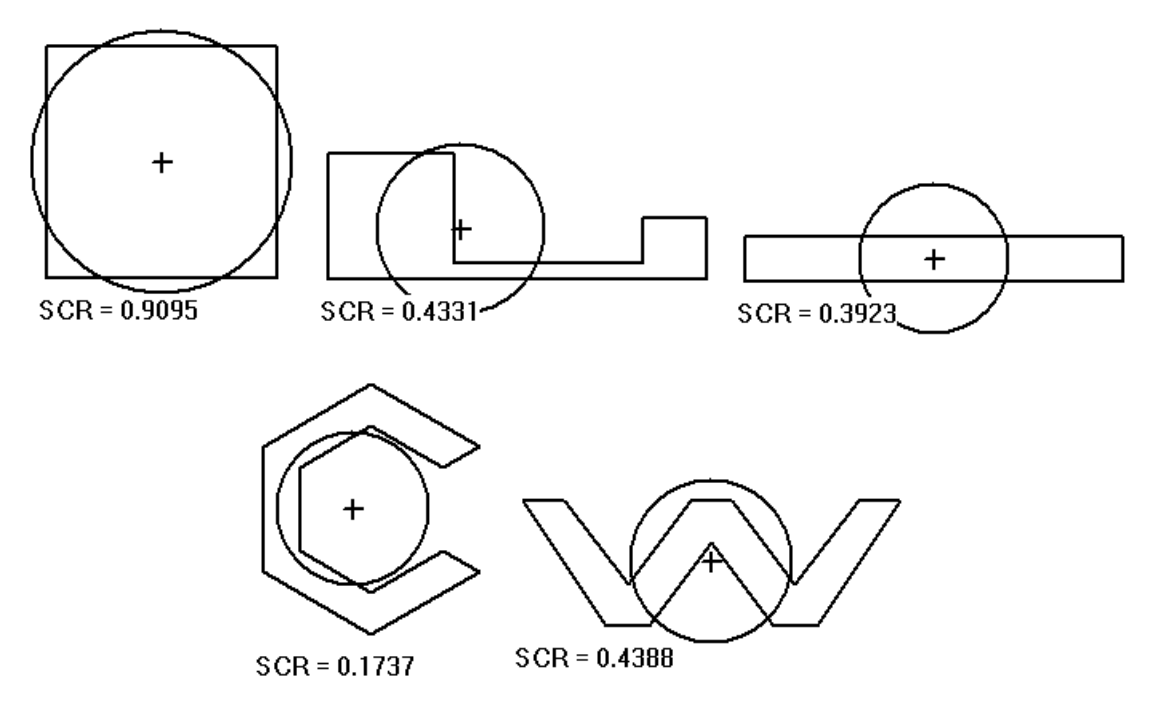시간 제한 메모리 제한 제출 정답 맞은 사람 정답 비율
1 초 512 MB 8 5 1 100.000%

## 문제

One of the criteria used in evaluating election districts is the compactness of the districts. Professor Fumblemore wants to investigate whether the roundness of election districts can be used to test for compactness. The simplified centered roundness SCR(R) of a plane region R is defined by:

• (x,y) is the centroid of R
• D is a disc centered at (x,y) with the same area as R
• SCR(R) = (Area of (R ∩ D)) / Area(R)

The simplified centered roundness will always be between 0 and 1. In practice, the region will be approximated by a polygon. Some examples: the cross (+) is the centroid of R:Write a program which takes as input the vertices of a polygon in counter-clockwise order (interior of the polygon is to the left of the boundary as you follow it) and finds the simplified centered roundness of the polygon.

## 입력

Input data consists of multiple lines of input. The first line contains the number N, (3 <= N <= 100) of vertices to follow. The first line is followed by (N+3)/4 additional lines consisting of 8 space separated floating point numbers representing the x and y coordinates of the vertices in order (x coordinate first). Each line, except perhaps the last, will contain the coordinates of 4 vertices.

## 출력

Output consists of a single line containing the simplified centered roundness of the polygon to 4 decimal places.

## 예제 입력 1

4
0 0 4 0 4 4 0 4


## 예제 출력 1

0.9095


## 예제 입력 2

8
0 0 12 0 12 2 10 2
10 0.5 4 0.5 4 4 0 4


## 예제 출력 2

0.4331